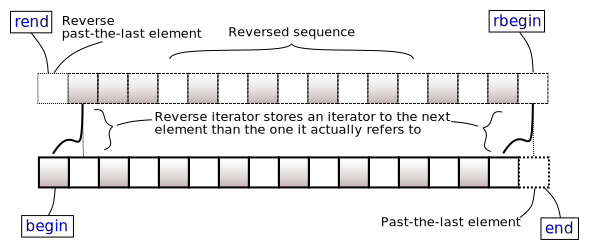/C++

# std::map<Key,T,Compare,Allocator>::rbegin, std::map<Key,T,Compare,Allocator>::crbegin

 (1) `reverse_iterator rbegin();` (until C++11) `reverse_iterator rbegin() noexcept;` (since C++11) (2) `const_reverse_iterator rbegin() const;` (until C++11) `const_reverse_iterator rbegin() const noexcept;` (since C++11) `const_reverse_iterator crbegin() const noexcept;` (3) (since C++11)

Returns a reverse iterator to the first element of the reversed `map`. It corresponds to the last element of the non-reversed `map`. If the `map` is empty, the returned iterator is equal to `rend()`.(none).

### Return value

Reverse iterator to the first element.

Constant.

### Example

```#include <iomanip>
#include <iostream>
#include <map>
#include <string_view>

int main()
{
const std::map<int, std::string_view> coins
{
{10, "dime"},
{100, "dollar"},
{50, "half dollar"},
{5, "nickel"},
{1, "penny"},
{25, "quarter"}
}; // initializer entries in name alphabetical order

std::cout << "US coins in circulation, largest to smallest denomination:\n";
for (auto it = coins.crbegin(); it != coins.crend(); ++it)
std::cout << std::setw(11) << it->second << " = ¢" << it->first << '\n';
}```

Output:

```US coins in circulation, largest to smallest denomination:
dollar = ¢100
half dollar = ¢50
quarter = ¢25
dime = ¢10
nickel = ¢5
penny = ¢1```